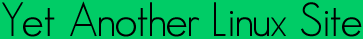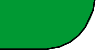Quick ?s
Cheat Sheets
Man Pages
The Lynx
Software
 ```MATH_ERROR(7) Linux Programmers Manual MATH_ERROR(7) SYNOPSIS #include #include #include NAME math_error - detecting errors from mathematical functions DESCRIPTION On error, many of the mathematical functions declared in return a NaN (not a number). However, rather than looking at the return value (which is not always possible) one can also check whether an error was signaled. There are two signaling mechanisms: the older one sets errno; the newer one uses the floating-point exception mecha nism (the use of feclearexcept(3) and fetestexcept(3), as outlined below) described in fenv(3). C99 and POSIX.1-2001 specify a math_errhandling identifier, which is supposed to indicate which of these two mechanisms is in use; the stan dards require that at least one be in use, but permit both to be avail able. Although glibc does not support this identifier, in practice it supports both mechanisms. A portable program that needs to check for an error from a mathematical function should set errno to zero, and make the following call feclearexcept(FE_ALL_EXCEPT); before calling a mathematical function. Upon return from the mathematical function, if errno is non-zero, or the following call (see fenv(3)) returns non-zero fetestexcept(FE_INVALID | FE_DIVBYZERO | FE_OVERFLOW | FE_UNDERFLOW); then an error occurred in the mathematical function. The error conditions that can occur for mathematical functions are described below. Domain Error A domain error occurs when a mathematical function is supplied with an argument whose value falls outside the domain for which the function is defined (e.g., giving a negative argument to log(3)). When a domain error occurs, errno is set to EDOM, and an "invalid" (FE_INVALID) floating-point exception is raised. Pole Error A pole error occurs when the mathematical result of a function is an exact infinity (e.g., the logarithm of 0 is negative infinity). When a pole error occurs, the function returns the (signed) value HUGE_VAL, HUGE_VALF, or HUGE_VALL, depending on whether the function result type is double, float, or long double. The sign of the result is that which is mathematically correct for the function. errno is set to ERANGE, and a "divide-by-zero" (FE_DIVBYZERO) floating-point exception is raised. Range Error A range error occurs when the magnitude of the function result means that it cannot be represented in the result type of the function. The return value of the function depends on whether the range error was an overflow or an underflow. A floating result overflows if the result is finite, but is too large to represented in the result type. When an overflow occurs, the func tion returns the value HUGE_VAL, HUGE_VALF, or HUGE_VALL, depending on whether the function result type is double, float, or long double. errno is set to ERANGE, and an "overflow" (FE_OVERFLOW) floating-point exception is raised. A floating result underflows if the result is too small to be repre sented in the result type. If an underflow occurs, a mathematical function typically returns 0.0 (C99 says a function shall return "an implementation-defined value whose magnitude is no greater than the smallest normalized positive number in the specified type"). errno may be set to ERANGE, and an "overflow" (FE_UNDERFLOW) floating-point exception may be raised. Some functions deliver a range error if the supplied argument value, or the correct function result, would be subnormal. A subnormal value is one that is non-zero, but with a magnitude that is so small that it cant be presented in normalized form (i.e., with a 1 in the most sig nificant bit of the significand). The representation of a subnormal number will contain one or more leading zeros in the significand. NOTES The math_errhandling identifier specified by C99 and POSIX.1-2001 is not supported. To avoid the complexities of using errno and fetestexcept(3) for error checking, it is often advised that one should instead check for bad argument values before each call. For example, the following code ensures that log(3)s argument is not a NaN and is not zero (a pole error) or less than zero (a domain error): double x, r; if (isnan(x) || islessequal(x, 0)) { /* Deal with NaN / pole error / domain error */ } r = log(x); The discussion on this page does not apply to the complex mathematical functions (i.e., those declared by ), which in general are not required to return errors by C99 and POSIX.1-2001. The gcc(1) -fno-math-errno option causes the executable to employ implementations of some mathematical functions that are faster than the standard implementations, but do not set errno on error. (The gcc(1) -ffast-math option also enables -fno-math-errno.) An error can still be tested for using fetestexcept(3). SEE ALSO gcc(1), errno(3), fenv(3), fpclassify(3), INFINITY(3), isgreater(3), matherr(3), nan(3) info libc COLOPHON This page is part of release 3.05 of the Linux man-pages project. A description of the project, and information about reporting bugs, can be found at http://www.kernel.org/doc/man-pages/. Linux 2008-07-21 MATH_ERROR(7) ```Yals.net is © 1999-2009 Crescendo Communications Sharing tech info on the web for more than a decade! This page was generated Thu Apr 30 17:05:31 2009